Work is related to force and the displacement over which it acts. Consider a constant force F acting on an object of mass m. The object undergoes a displacement d in the positive x-direction (see fig. 1)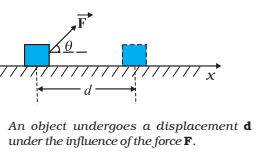Fig. 1

The work done by the force is defined to be the product of the component of the force in the direction of the displacement and the magnitude of this displacement.

Thus, W = (F cosθ) d = F. d

### Work Done by a Variable Force:

It is the variable force, which is more commonly encountered. Fig. 1 is a plot of a varying force in one dimension. If the displacement Δx is small, we can take the force F (x) is approximately constant and the work done is then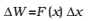This is illustrated in Fig.2 (a). Adding successive rectangular areas in Fig.2 (a) we get the total work done aswhere the summation is from the initial position xi to the final position xf .

If the displacements are allowed to approach zero, then the number of terms in the sum increases without limit, but the sum approaches a definite value equal to the area under the curve in Fig.2 (b).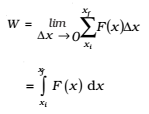where ‘lim’ stands for the limit of the sum when Δx tends to zero. Thus, for a varying force, the work done can be expressed as a definite integral of force over displacement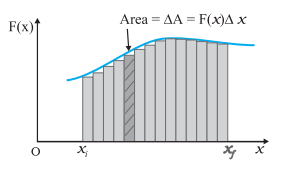Fig.2 (a)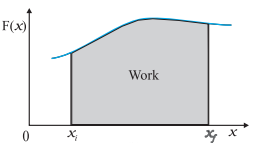Fig.2 (b)

Fig.1(a) The shaded rectangle represents the work done by the varying force F(x), over the small displacement Δx, ΔW = F(x)Δx. Fig.1(b) adding the areas of all the rectangles we find that for Δx →0, the area under the curve is exactly equal to the work done by F(x).